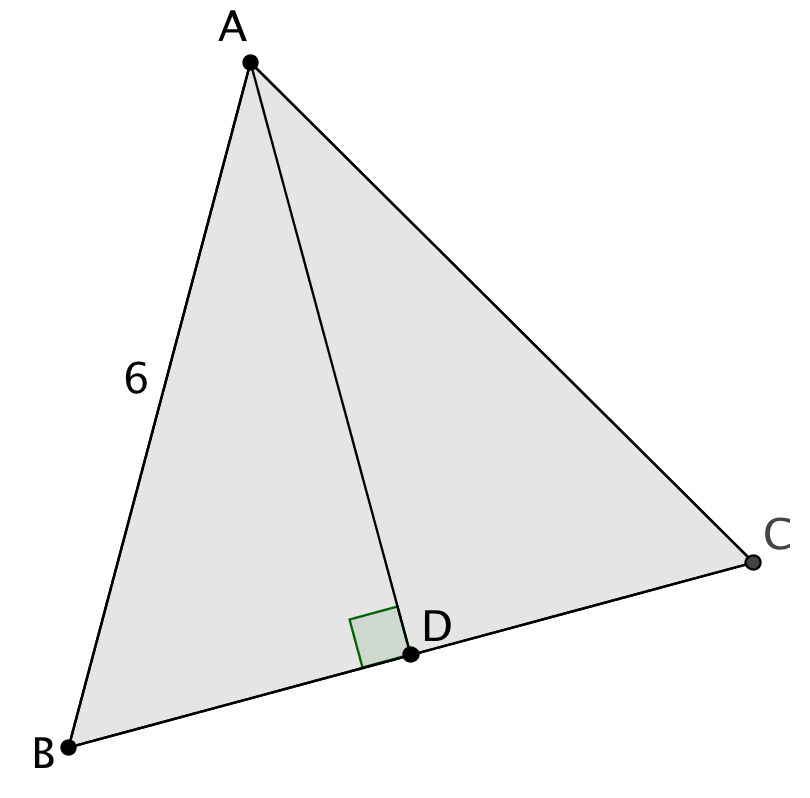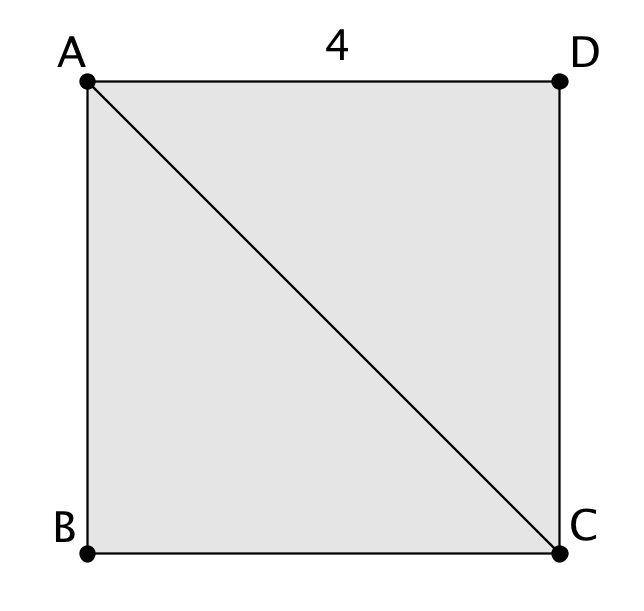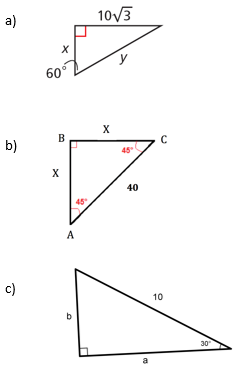# Right Triangles and Trigonometry

Lesson 3

Math

Unit 4

Lesson 3 of 19

## Objective

Define the relationship between side lengths of special right triangles.

## Common Core Standards

### Core Standards

• G.SRT.B.4 — Prove theorems about triangles. Theorems include: a line parallel to one side of a triangle divides the other two proportionally, and conversely; the Pythagorean Theorem proved using triangle similarity.
• G.SRT.B.5 — Use congruence and similarity criteria for triangles to solve problems and to prove relationships in geometric figures.

• G.CO.C.10

## Criteria for Success

1. Derive the relationship between side lengths of right triangles with measures of 45°-45°-90° from a square with a diagonal drawn, the properties of base angles of isosceles triangles, and the Pythagorean theorem.
2. Define the general algebraic relationship between all 45°-45°-90° triangles, citing similarity as the reason for why this relationship can be extrapolated.
3. Derive the relationship between side lengths of right triangles with angle measures of 30°-60°-90° from an equilateral triangle with an angle bisector drawn from a vertex, and the Pythagorean theorem.
4. Define the general algebraic relationship between all 30°-60°-90° triangles, citing similarity as the reason for why this relationship can be extrapolated.
5. Algebraically find the relationship for any side length of special right triangles.

## Tips for Teachers

• The following resource is helpful to understand how special right triangles fit in to the common core standards: Mathematical Musings, “Special Right Triangles
• At this point, students are NOT expected to be able to rationalize the denominator or multiply radicals when finding the side lengths of special right triangles. This skill will be developed in the next lesson.
Fishtank Plus

Unlock features to optimize your prep time, plan engaging lessons, and monitor student progress.

## Anchor Problems

### Problem 1

Below is an equilateral triangle with a perpendicular bisector drawn from vertex $$A$$

Based on this information, what else do you know about the resultant triangles?### Problem 2

Below is a square with diagonal $${\overline {AC}}$$.

Based on this information, what else do you know about the resultant triangles?## Problem Set

The following resources include problems and activities aligned to the objective of the lesson that can be used to create your own problem set.

• Include problems where students are asked to find the actual values of missing legs and hypotenuse of special right triangles.
• Include problems where students identify error of relationships between side lengths and hypotenuse of special right triangles.
• Include problems that require students to explain their thinking and describe from where the two special right triangles are derived.

Find the missing side lengths. Show your reasoning.Lesson 2

Lesson 4

## Lesson Map

Topic A: Right Triangle Properties and Side-Length Relationships

Topic B: Right Triangle Trigonometry

Topic C: Applications of Right Triangle Trigonometry

Topic D: The Unit Circle

Topic E: Trigonometric Ratios in Non-Right Triangles